Скачать презентацию Chapter 4 Stock Bond Valuation Professor XXXXX

26ce7a60e6987c9eaf0600b1f6dfd303.ppt

• Количество слайдов: 42Chapter 4 Stock & Bond Valuation Professor XXXXX Course Name / Number © 2007 Thomson South-WesternValuation Fundamentals q The greater the uncertainty about an asset’s future benefits, the higher the discount rate investors will apply when discounting those benefits to the present. q The valuation process links an asset’s risk and return to determine its price. 22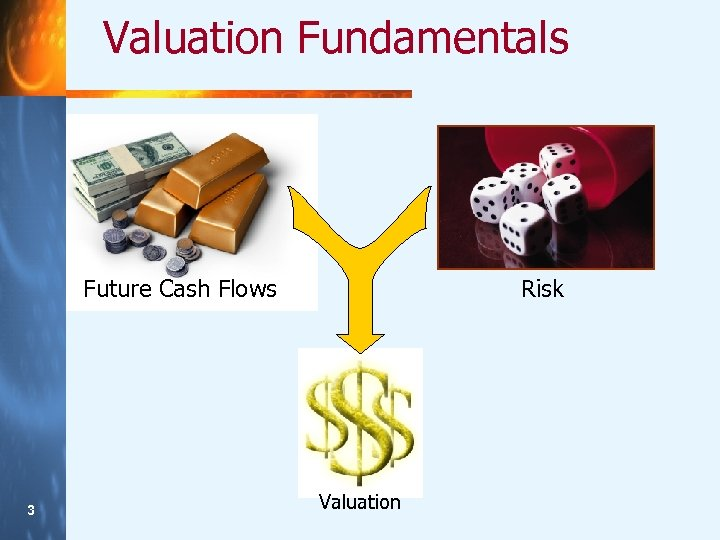Valuation Fundamentals Future Cash Flows 33 Risk Valuation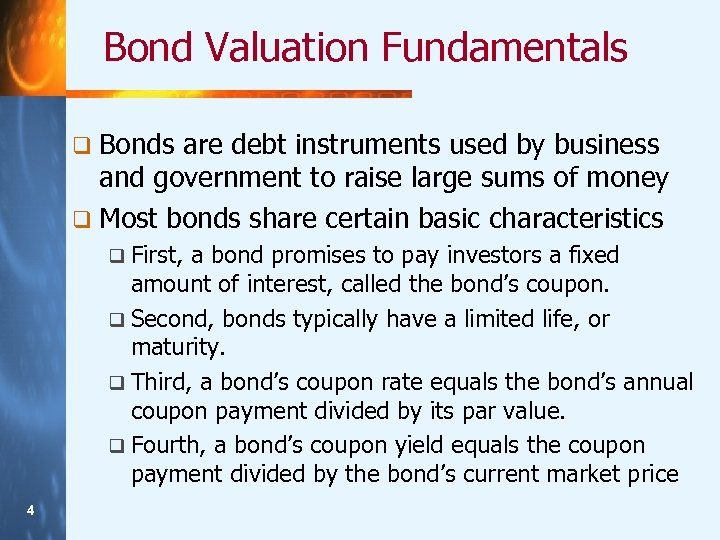Bond Valuation Fundamentals q Bonds are debt instruments used by business and government to raise large sums of money q Most bonds share certain basic characteristics q First, a bond promises to pay investors a fixed amount of interest, called the bond’s coupon. q Second, bonds typically have a limited life, or maturity. q Third, a bond’s coupon rate equals the bond’s annual coupon payment divided by its par value. q Fourth, a bond’s coupon yield equals the coupon payment divided by the bond’s current market price 44Valuation Fundamentals Present Value of Future Cash Flows Link Risk & Return Expected Return on Assets Valuation 55The Basic Valuation Model P 0 = Price of asset at time 0 (today) q CFt = cash flow expected at time t q r = discount rate (reflecting asset’s risk) q n = number of discounting periods (usually years) q 66 This model can express the price of any asset at t = 0 mathematically.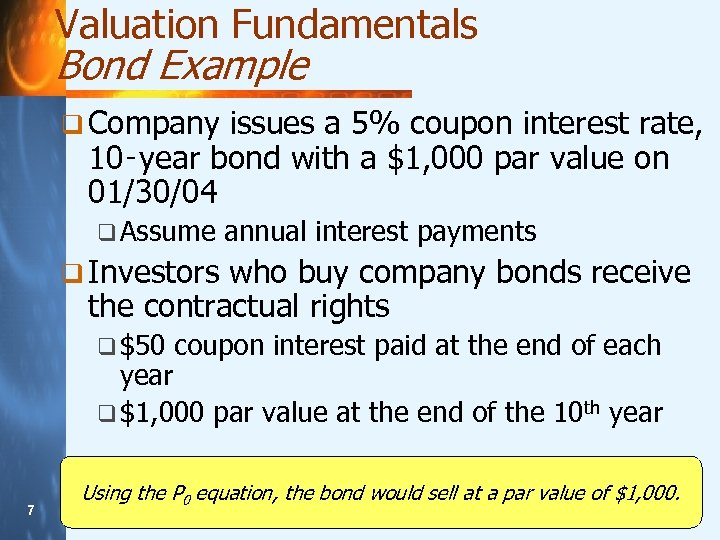Valuation Fundamentals Bond Example q Company issues a 5% coupon interest rate, 10‑year bond with a \$1, 000 par value on 01/30/04 q Assume annual interest payments q Investors who buy company bonds receive the contractual rights q \$50 coupon interest paid at the end of each year q \$1, 000 par value at the end of the 10 th year 77 Using the P 0 equation, the bond would sell at a par value of \$1, 000.Bonds: Premiums & Discounts What Happens to Bond Values if the Required Return Is Not Equal to the Coupon Rate? The bond's value will differ from its par value R > Coupon Interest Rate = DISCOUNT R < Coupon Interest Rate 88 P 0 < par value P 0 > par value = PREMIUMThe Basic Equation (Assuming Annual Interest) q Cash flows include two components: the annual coupon, C, which equals the stated coupon rate, i, multiplied by M, the par value (that is, C i M), received for each of the n years q (2) the par value, M, received at maturity in n years q (1) 99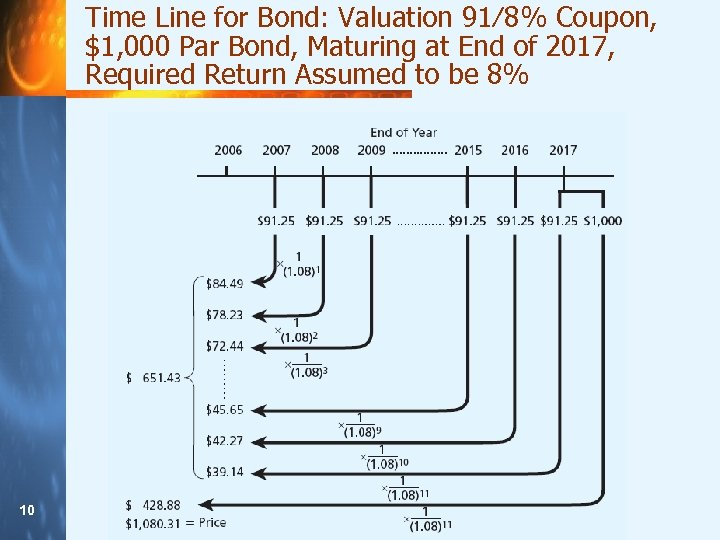Time Line for Bond: Valuation 91⁄8% Coupon, \$1, 000 Par Bond, Maturing at End of 2017, Required Return Assumed to be 8% 10 10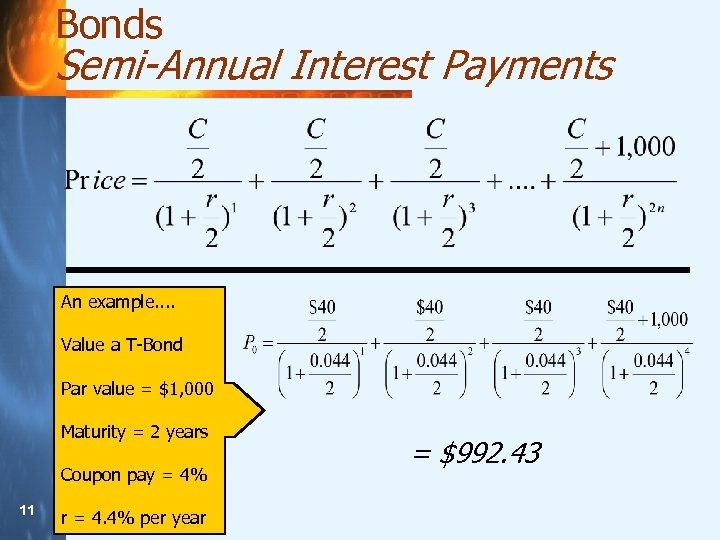Bonds Semi-Annual Interest Payments An example. . Value a T-Bond Par value = \$1, 000 Maturity = 2 years Coupon pay = 4% 11 11 r = 4. 4% per year = \$992. 43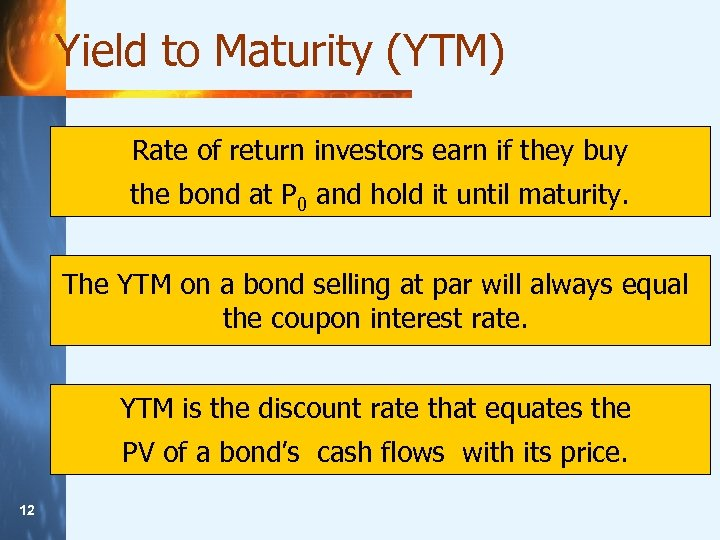Yield to Maturity (YTM) Rate of return investors earn if they buy the bond at P 0 and hold it until maturity. The YTM on a bond selling at par will always equal the coupon interest rate. YTM is the discount rate that equates the PV of a bond’s cash flows with its price. 12 12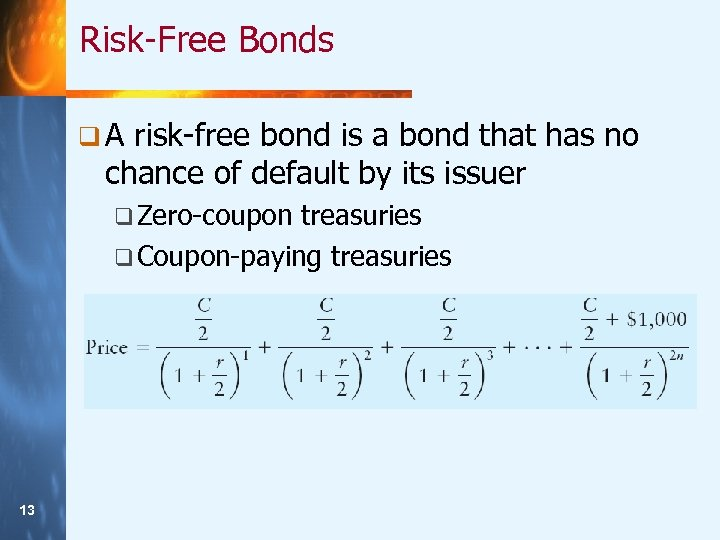Risk-Free Bonds q. A risk-free bond is a bond that has no chance of default by its issuer q Zero-coupon treasuries q Coupon-paying treasuries 13 13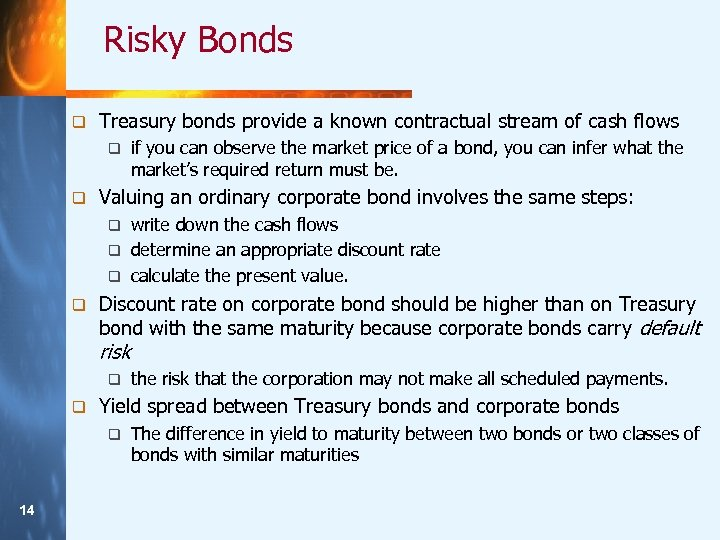Risky Bonds q Treasury bonds provide a known contractual stream of cash flows q q if you can observe the market price of a bond, you can infer what the market’s required return must be. Valuing an ordinary corporate bond involves the same steps: write down the cash flows q determine an appropriate discount rate q calculate the present value. q q Discount rate on corporate bond should be higher than on Treasury bond with the same maturity because corporate bonds carry default risk q q Yield spread between Treasury bonds and corporate bonds q 14 14 the risk that the corporation may not make all scheduled payments. The difference in yield to maturity between two bonds or two classes of bonds with similar maturities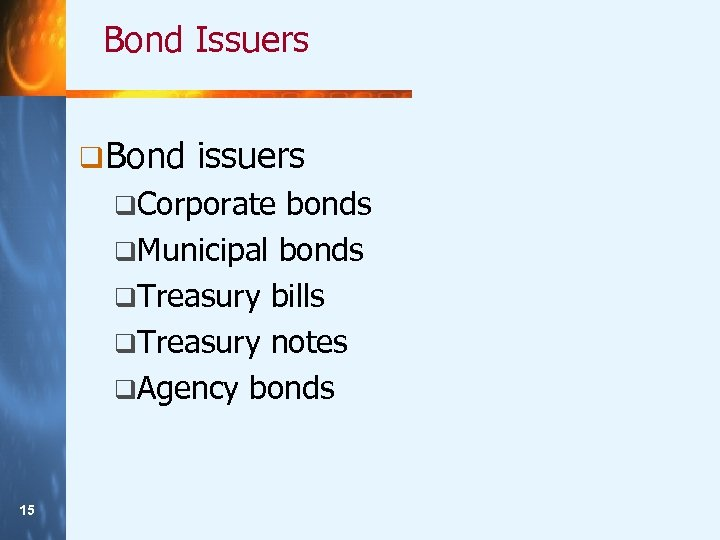Bond Issuers q Bond issuers q. Corporate bonds q. Municipal bonds q. Treasury bills q. Treasury notes q. Agency bonds 15 15Bond Ratings q Bond ratings q. Moody’s q. Standard q. Fitch 16 16 & Poor’s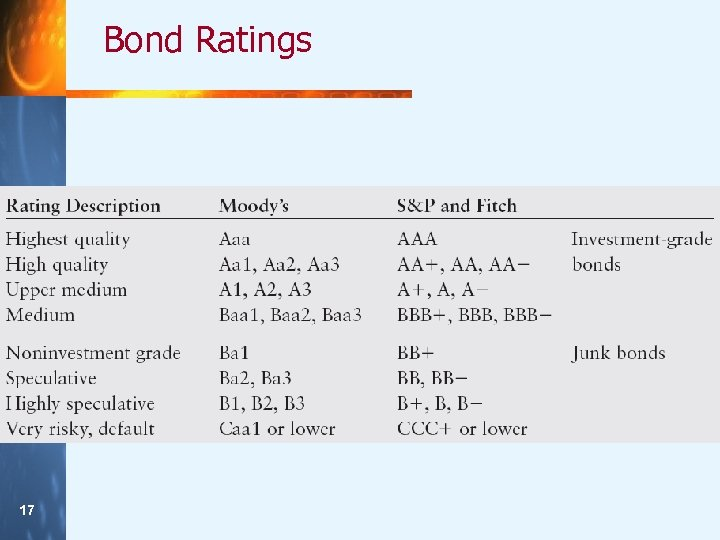Bond Ratings 17 17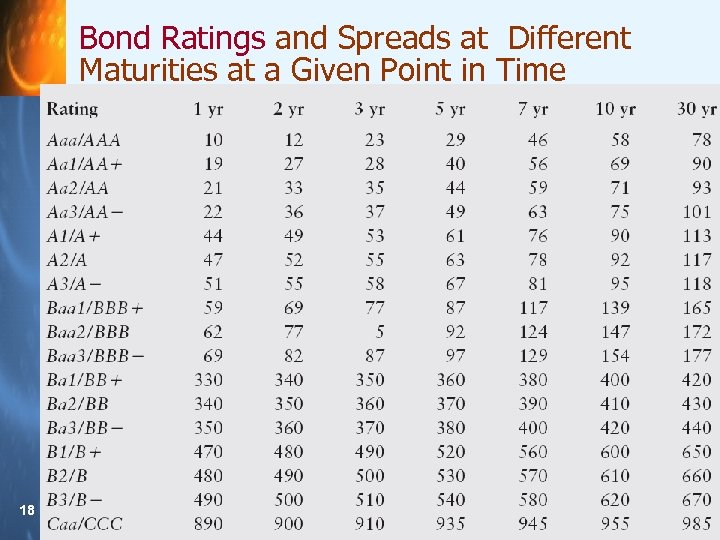Bond Ratings and Spreads at Different Maturities at a Given Point in Time 18 18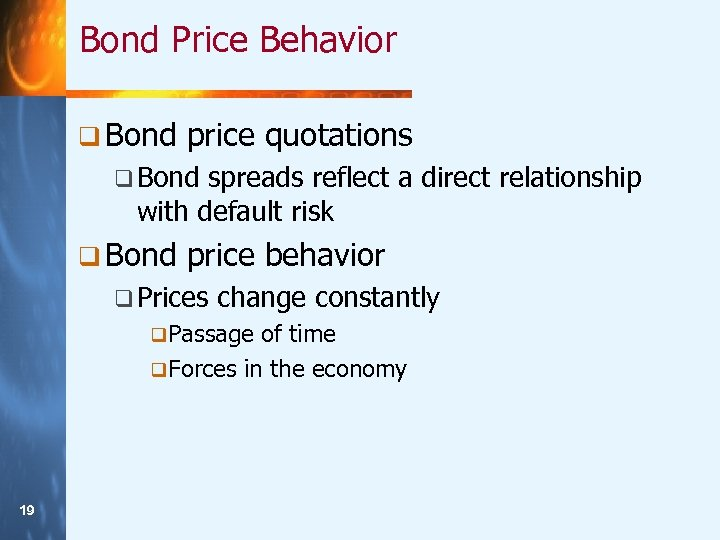Bond Price Behavior q Bond price quotations q Bond spreads reflect a direct relationship with default risk q Bond price behavior q Prices change constantly q. Passage of time q. Forces in the economy 19 19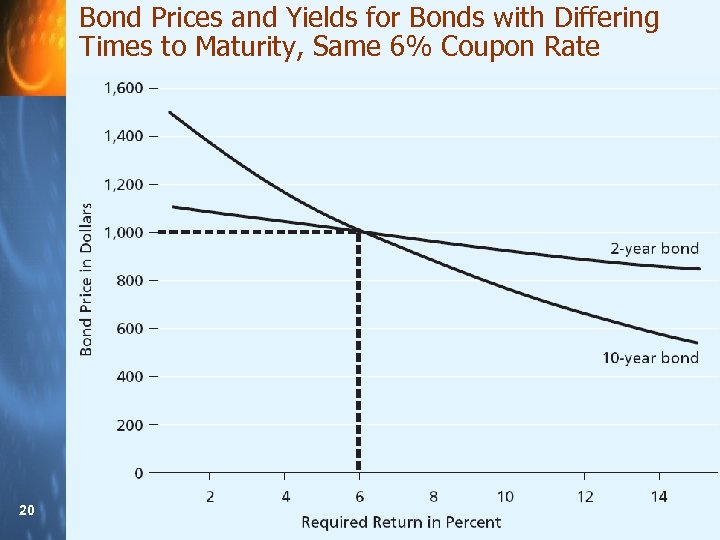Bond Prices and Yields for Bonds with Differing Times to Maturity, Same 6% Coupon Rate 20 20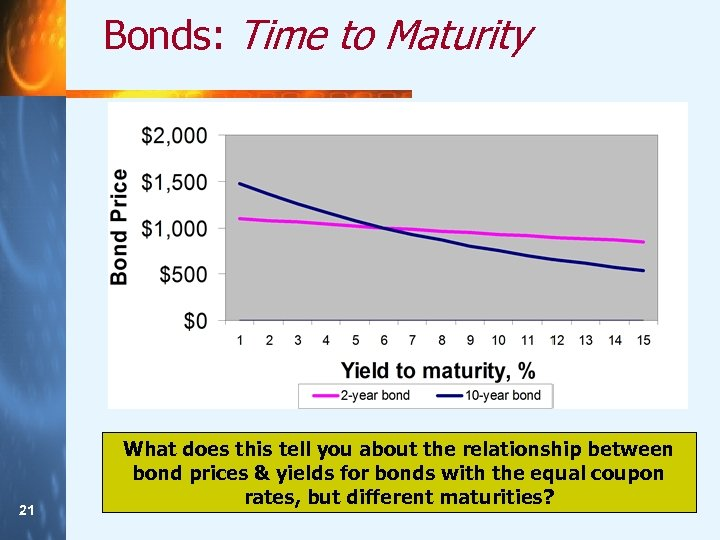Bonds: Time to Maturity 21 21 What does this tell you about the relationship between bond prices & yields for bonds with the equal coupon rates, but different maturities?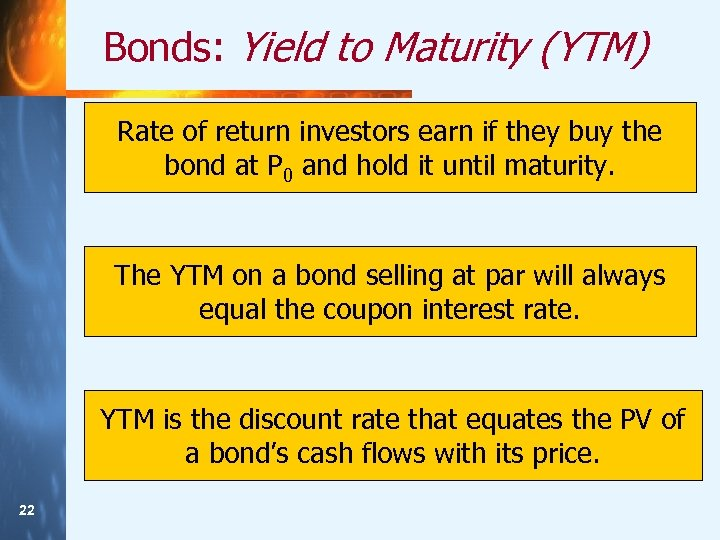Bonds: Yield to Maturity (YTM) Rate of return investors earn if they buy the bond at P 0 and hold it until maturity. The YTM on a bond selling at par will always equal the coupon interest rate. YTM is the discount rate that equates the PV of a bond’s cash flows with its price. 22 22Evaluating the Yield Curve q Yields vary with maturity. q Yields offered by bonds must be sufficient to offer investors a positive real return. q The real return on an investment approximately equals the difference between its stated or nominal return and the inflation rate. q The shape of the yield curve can change over time. q Research shows the yield curve works well as a predictor of economic activity, in the United States and other large industrialized economies. 23 23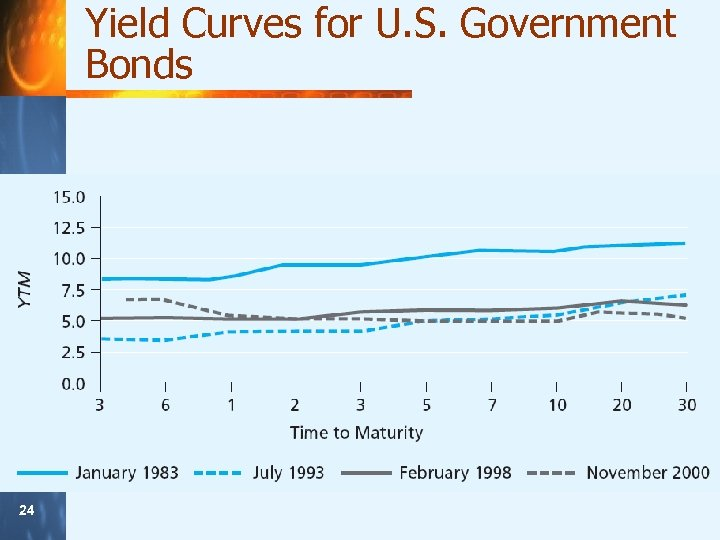Yield Curves for U. S. Government Bonds 24 24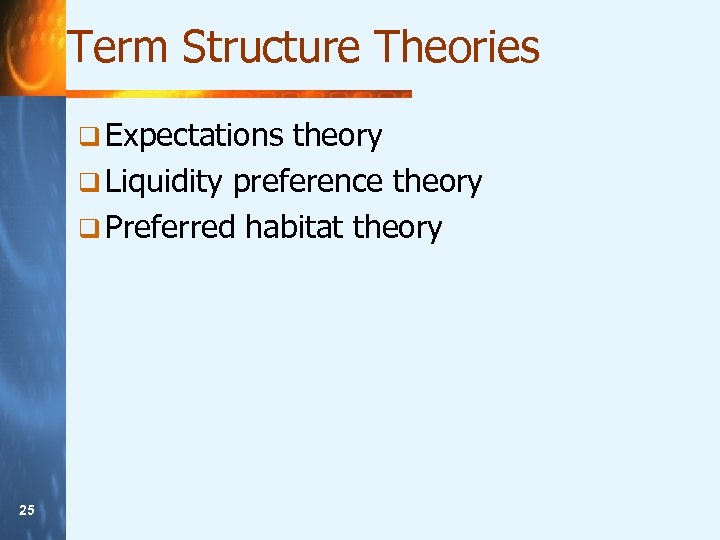Term Structure Theories q Expectations theory q Liquidity preference theory q Preferred habitat theory 25 25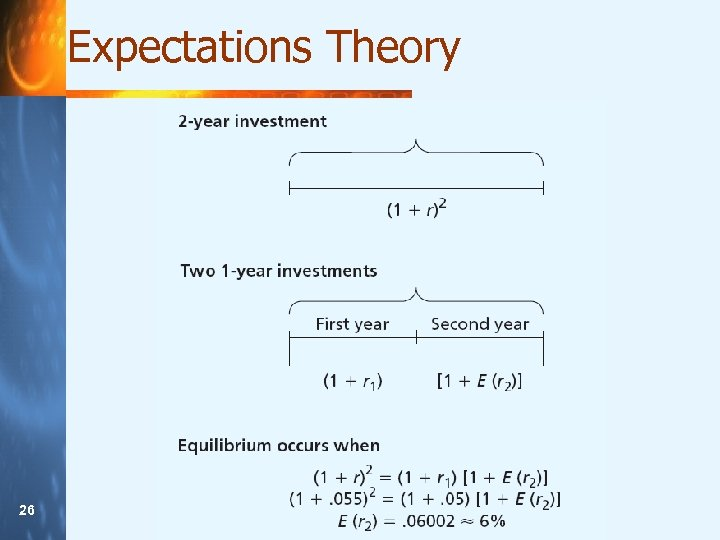Expectations Theory 26 26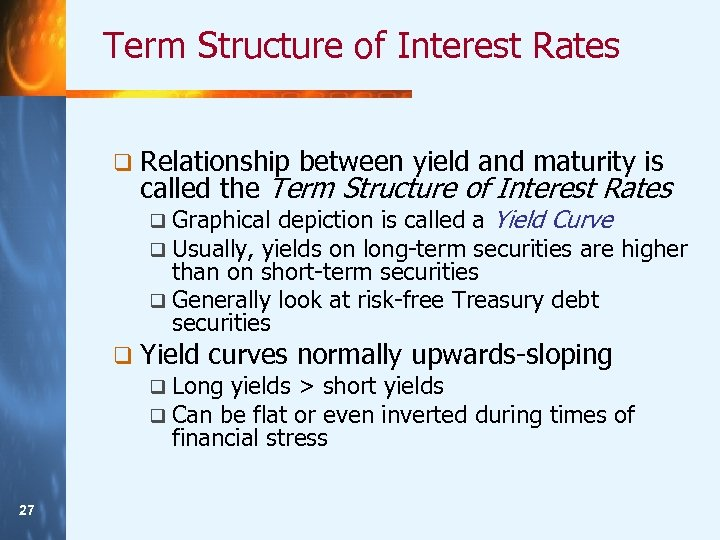Term Structure of Interest Rates q Relationship between yield and maturity is called the Term Structure of Interest Rates a Yield Curve securities are higher than on short-term securities q Generally look at risk-free Treasury debt securities q Graphical depiction is called q Usually, yields on long-term q Yield curves normally upwards-sloping q Long yields > short yields q Can be flat or even inverted during times of financial stress 27 27Stock Valuation: Preferred Stock Preferred stock is an equity security that is expected to pay a fixed annual dividend for its life PS 0 = Preferred stock’s value DP = preferred dividend rp = required rate of return An example: A share of preferred stock pays \$2. 3 per share annual dividend and with a required return of 11% PS 0= 28 28 DP rp = \$2. 30 0. 11 = \$20. 90 / share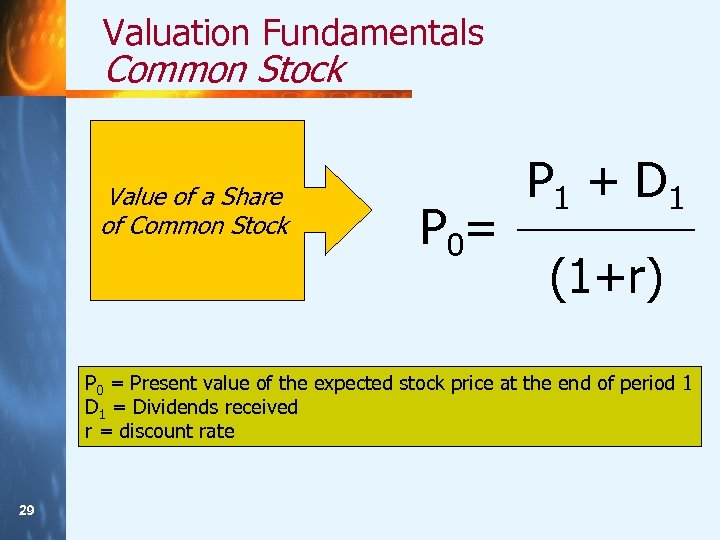Valuation Fundamentals Common Stock Value of a Share of Common Stock P 0 = P 1 + D 1 (1+r) P 0 = Present value of the expected stock price at the end of period 1 D 1 = Dividends received r = discount rate 29 29Valuation Fundamentals: Common Stock q But how is P 1 determined? q This is the PV of expected stock price P 2, plus dividend at time 2 q P 2 is the PV of P 3 plus dividend at time 3, etc. . . q Repeating this logic over and over, you find that today’s price equals PV of the entire dividend stream the stock will pay in the future 30 30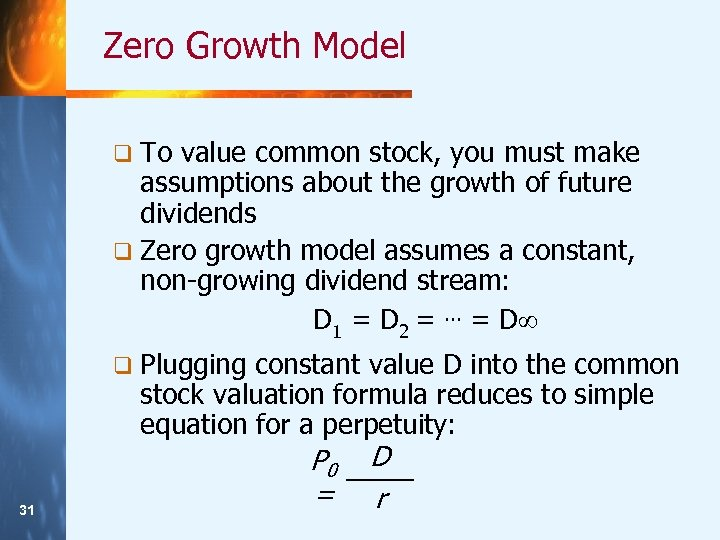Zero Growth Model q To value common stock, you must make assumptions about the growth of future dividends q Zero growth model assumes a constant, non-growing dividend stream: D 1 = D 2 =. . . = D q Plugging constant value D into the common stock valuation formula reduces to simple equation for a perpetuity: 31 31 P 0 = D r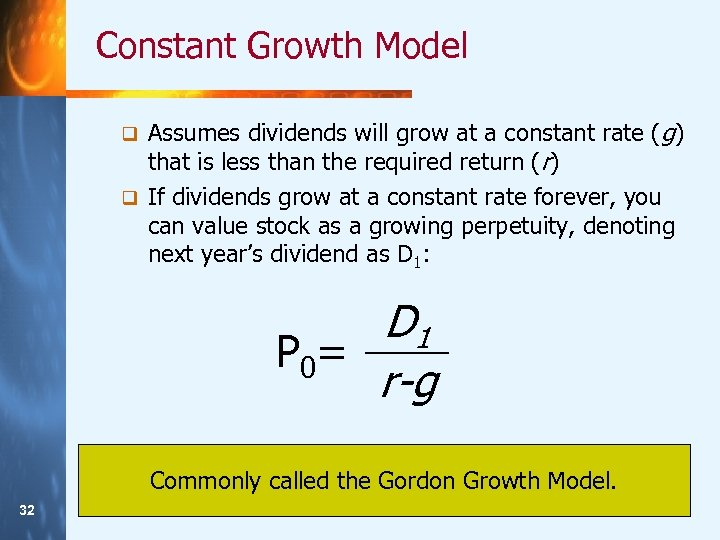Constant Growth Model Assumes dividends will grow at a constant rate (g) that is less than the required return (r) q If dividends grow at a constant rate forever, you can value stock as a growing perpetuity, denoting next year’s dividend as D 1: q D 1 P 0 = r-g Commonly called the Gordon Growth Model. 32 32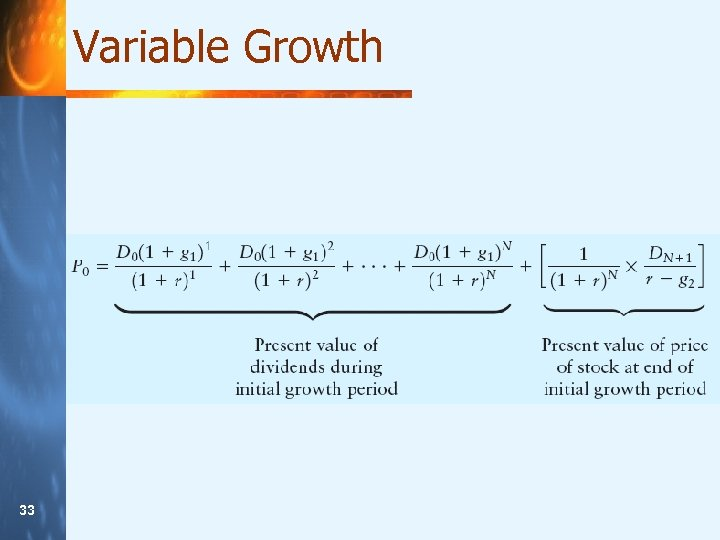Variable Growth 33 33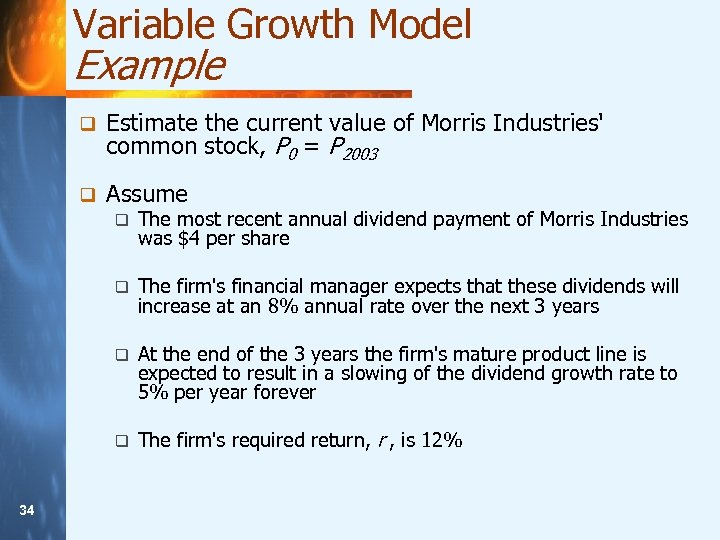Variable Growth Model Example q Estimate the current value of Morris Industries' common stock, P 0 = P 2003 q Assume q q The firm's financial manager expects that these dividends will increase at an 8% annual rate over the next 3 years q At the end of the 3 years the firm's mature product line is expected to result in a slowing of the dividend growth rate to 5% per year forever q 34 34 The most recent annual dividend payment of Morris Industries was \$4 per share The firm's required return, r , is 12%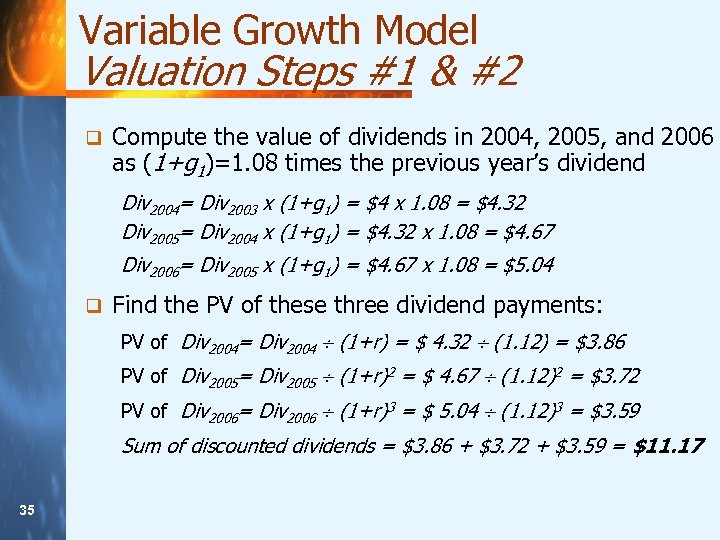Variable Growth Model Valuation Steps #1 & #2 q Compute the value of dividends in 2004, 2005, and 2006 as (1+g 1)=1. 08 times the previous year’s dividend Div 2004= Div 2003 x (1+g 1) = \$4 x 1. 08 = \$4. 32 Div 2005= Div 2004 x (1+g 1) = \$4. 32 x 1. 08 = \$4. 67 Div 2006= Div 2005 x (1+g 1) = \$4. 67 x 1. 08 = \$5. 04 q Find the PV of these three dividend payments: PV of Div 2004= Div 2004 (1+r) = \$ 4. 32 (1. 12) = \$3. 86 PV of Div 2005= Div 2005 (1+r)2 = \$ 4. 67 (1. 12)2 = \$3. 72 PV of Div 2006= Div 2006 (1+r)3 = \$ 5. 04 (1. 12)3 = \$3. 59 Sum of discounted dividends = \$3. 86 + \$3. 72 + \$3. 59 = \$11. 17 35 35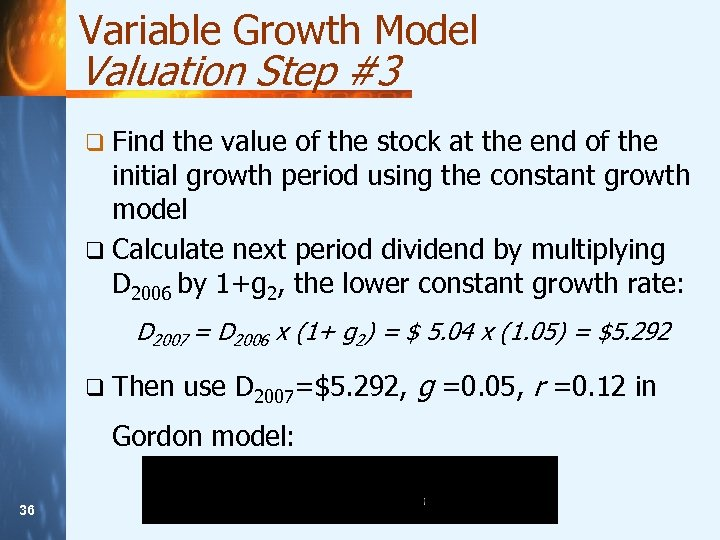Variable Growth Model Valuation Step #3 q Find the value of the stock at the end of the initial growth period using the constant growth model q Calculate next period dividend by multiplying D 2006 by 1+g 2, the lower constant growth rate: D 2007 = D 2006 x (1+ g 2) = \$ 5. 04 x (1. 05) = \$5. 292 q Then use D 2007=\$5. 292, g =0. 05, r =0. 12 in Gordon model: 36 36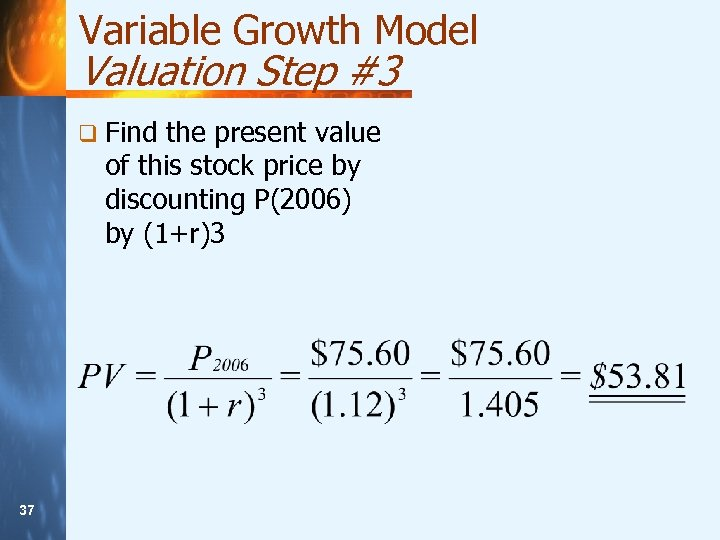Variable Growth Model Valuation Step #3 q Find the present value of this stock price by discounting P(2006) by (1+r)3 37 37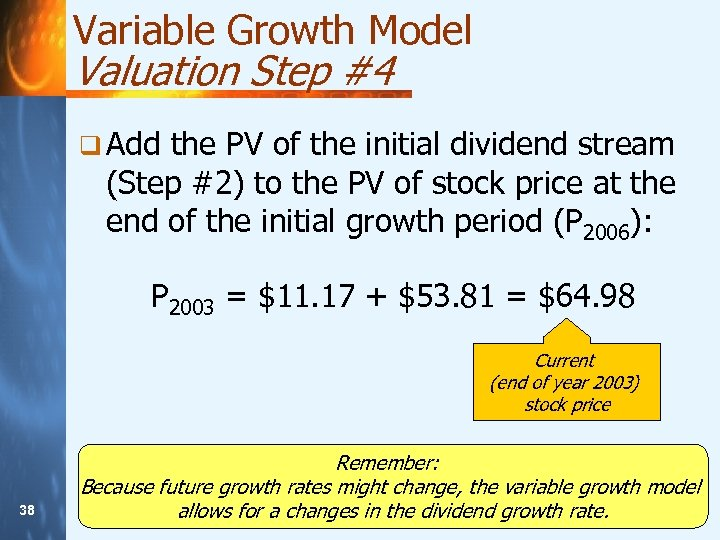Variable Growth Model Valuation Step #4 q Add the PV of the initial dividend stream (Step #2) to the PV of stock price at the end of the initial growth period (P 2006): P 2003 = \$11. 17 + \$53. 81 = \$64. 98 Current (end of year 2003) stock price 38 38 Remember: Because future growth rates might change, the variable growth model allows for a changes in the dividend growth rate.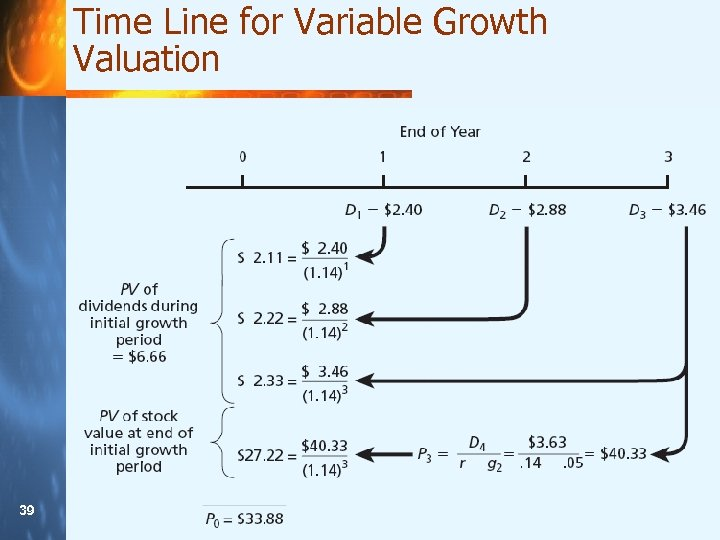Time Line for Variable Growth Valuation 39 39Free Cash Flow Approach by asking, what is the total operating cash flow (OCF) generated by a firm? q Next subtract from the firm’s operating cash flow the amount needed to fund new investments in both fixed assets and current assets. q The difference is total free cash flow (FCF). q Begin q Represents the cash amount a firm could distribute to investors after meeting all its other obligations 40 40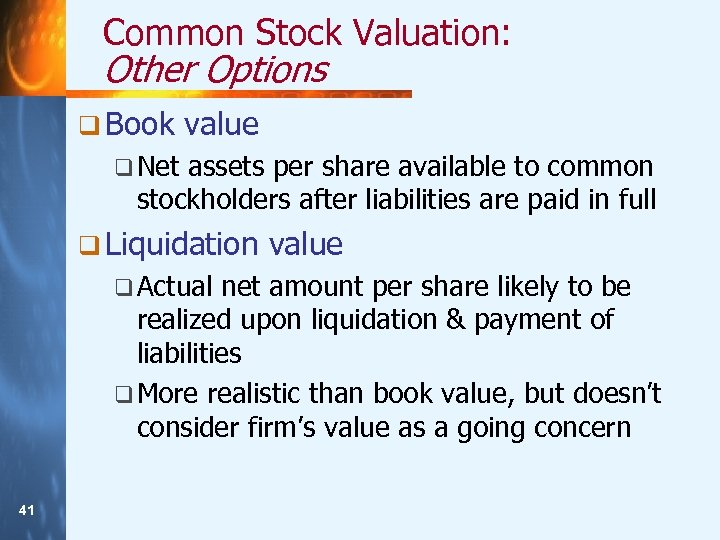Common Stock Valuation: Other Options q Book value q Net assets per share available to common stockholders after liabilities are paid in full q Liquidation q Actual value net amount per share likely to be realized upon liquidation & payment of liabilities q More realistic than book value, but doesn’t consider firm’s value as a going concern 41 41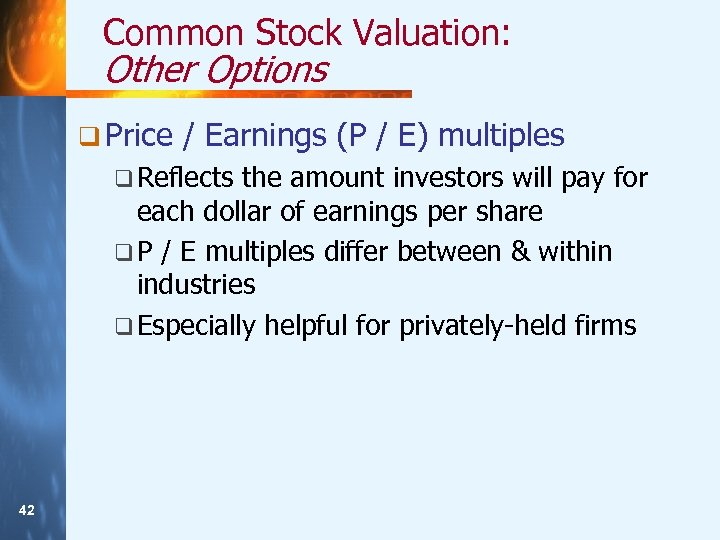Common Stock Valuation: Other Options q Price / Earnings (P / E) multiples q Reflects the amount investors will pay for each dollar of earnings per share q P / E multiples differ between & within industries q Especially helpful for privately-held firms 42 42